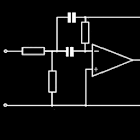Linear convolution Theorem

• SEARCH TYPE

All Android applications categories

All Android games categories# Linear convolution Theorem

by: 11 9

9 Users
rating

## Screenshots

Description

This is an app for those who use the linear convolution theorem. It will directly give you the result of the problem as you enter your X and Y values.
The data entered is in a very easy and interactive way, and even calculations for the element at the zeroth position are made.

Tags: linear convolution , convolution in , convolution theorem in , convolution 안드로이드 , test of linear convolution , 안드로이드 convolution

## Users review

from 11 reviews

"Awesome"

9